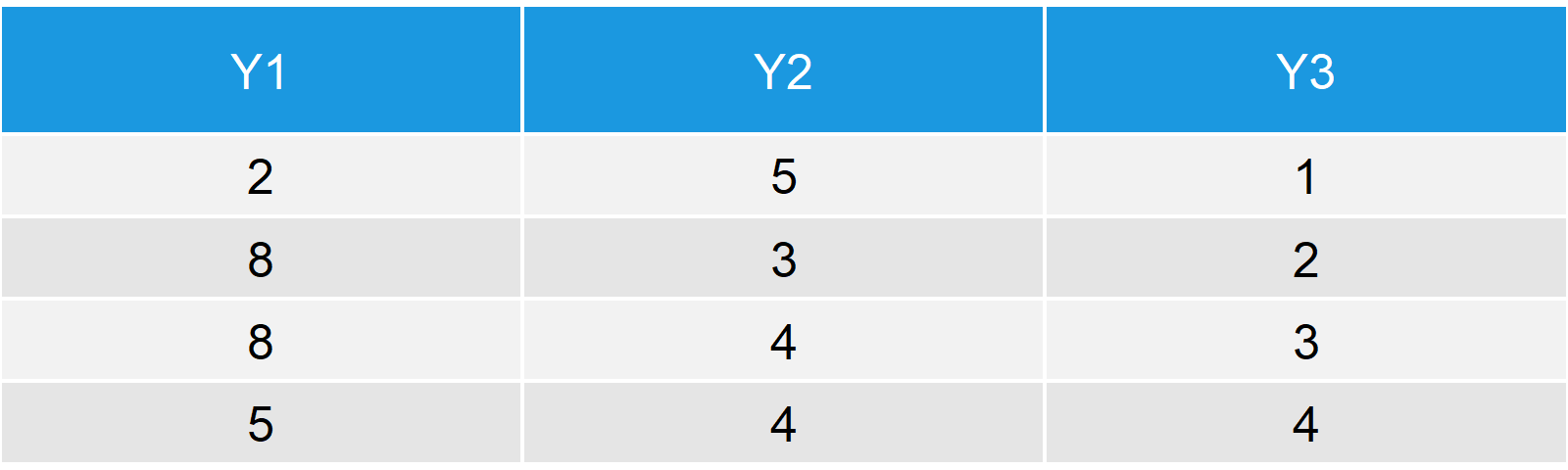# The setdiff R Function (3 Example Codes)

Basic R Syntax:

`setdiff(x, y)`

The R function setdiff indicates which elements of a vector or data frame X are not existent in a vector or data frame Y. The previous code illustrates how to use setdiff in R.

In the following article, I’ll show you 3 examples for the usage of the setdiff command in R. Let’s start right away…

## Example 1: Apply setdiff to Numeric Vectors in R

Let’s first create two example vectors in R:

```x <- c(1, 5, 3, 1, 2, 9)              # Example vector 1
y <- c(7, 6, 8, 9, 5, 5, 5, 3)        # Example vector 2```

Now, let’s apply the setdiff function to these two vectors:

```setdiff(x, y)                         # Apply setdiff function in R
# 1 2```

The output in the RStudio console is 1 and 2 – These two values do appear in X, but they do not appear in Y.

Note: If you use setdiff the opposite way, i.e. Y and X exchanged, then you receive a different result. Let’s check what we get:

```setdiff(y, x)                         # Opposite ordering of X and Y
# 7 6 8```

If we use the setdiff R function the other way around, we receive all values that appear in Y, but not in X. Be careful with the ordering!

By the way: I have recently published a video, in which I’m explaing the previous R code in some more detail. You can have a look at the video below:

Please accept YouTube cookies to play this video. By accepting you will be accessing content from YouTube, a service provided by an external third party.If you accept this notice, your choice will be saved and the page will refresh.

## Example 2: Use the setdiff Command for Character Vectors

Again, let’s create some example vectors in R – This time character (or string) vectors.

```x_char <- c("A", "D", "Hello", "RRR") # Character vector 1
y_char <- c("A", "B", "RRR")          # Character vector 2```

If we apply the setdiff R command, we get the character values back, that are existent in the X vector, but not in the Y vector:

```setdiff(x_char, y_char)               # Apply setdiff function in R
# "D"     "Hello"```

In case of our two example vectors, we get the strings D and Hello.

## Example 3: setdiff Between R Data Frames

So far, the application of setdiff was quite straightforward. However, the setdiff function can also be used to examine the difference between two data.frames, i.e. multiple columns at once. Consider the following example data:

```data1 <- data.frame(x1 = c(1, 4, 8, 1),    # Data Frame 1
x2 = c(5, 5, 1, 4),
x3 = c(1, 2, 3, 4))
data2 <- data.frame(y1 = c(2, 8, 8, 5),    # Data Frame 2
y2 = c(5, 3, 4, 4),
y3 = c(1, 2, 3, 4))```Table 1: First Example Data Frame.Table 2: Second Example Data Frame.

Let’s apply setdiff to these two data tables:

```setdiff(data1, data2)                      # Apply setdiff to data frames
# x1 x2
#  1  5
#  4  5
#  8  1
#  1  4```

The setdiff R function returns only the first two columns of our first data matrix data1. What happened to the third column?

Check carefully: X3 and Y3 are identical! For that reason, setdiff returns only the first two columns – The columns that are different.

However, the function returns all rows of these two columns, even though some values of X1 and X2 do also exist in Y1 and Y2.

## Video Application of setdiff: How to Find a Mismatch in R

The video below of the YouTube channel Xperimental Learning provides you with further examples for the application on setdiff in R:

Please accept YouTube cookies to play this video. By accepting you will be accessing content from YouTube, a service provided by an external third party.If you accept this notice, your choice will be saved and the page will refresh.

Subscribe to the Statistics Globe Newsletter

•Kashish
May 18, 2020 10:41 am

I used your example employing the setdiff() function on the dataframes. However, in my case, it was not executed and it gave the following error:

Error: not compatible:
– Cols in y but not x: `y3`, `y1`, `y2`.
– Cols in x but not y: `x3`, `x1`, `x2`.

•May 18, 2020 11:39 am

Hi Kashish,

Could you send the exact R code you are using? Without the code, it’s difficult to spot the issue.

Regards,

Joachim

•Unnikrishnan C
January 6, 2021 4:13 pm

Your method of imparting R knowledge is very simple and lucid and deeply satisfying to the learner.

•January 6, 2021 4:39 pm

Hey Unnikrishnan,

Thanks a lot for this awesome feedback. I’m glad to hear that you like my content! 🙂

Regards, Joachim

•Mindy
April 28, 2021 10:48 pm

Thanks for the simple, yet thorough explanation of setdiff(). Very helpful to get some actual concrete examples explained for R functions. Keep documenting please!

•April 29, 2021 5:46 am

Hey Mindy,

Thanks a lot for this great feedback! I’m glad to hear that the tutorial helped 🙂

Regards

Joachim

•Carolina
May 22, 2021 1:01 am

Hi, What could happen if in the first vector a value twice and in the second vector it only appears once. The function is able to tell that difference? What function could I use if I want you to notice these kinds of differences in the data?

•May 25, 2021 6:43 am

Hey Carolina,

Maybe you could use the table() function for this? You could apply the table function once to the first column and once to the second column. This way you would see the count of each value in each of the columns.

I hope that helps!

Joachim

••August 6, 2021 6:18 am